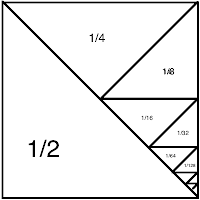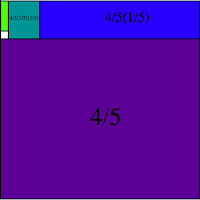## Monday, June 25, 2012

### Zeno's Paradox and Why It Annoys Me

I have always been greatly annoyed by Zeno's paradoxes.

The reason why is due mostly to my stubborn pride at being ignored when I'm right.  When I was in 10th grade trig, we learned Zeno's paradox of Achilles and the Tortoise.  The problem so presented is an extremely simple algebraic equation, immediately solvable to anyone who has finished high school.

So in grade school, when I was taught this "paradox", I did solve it algebraically, almost before my teacher had finished reading it from the book, and I told her the answer, and she sort of gave me this exasperated smile and said "Yes, I know, but don't think about it that way."  And ever since, mention of this paradox as anything other than an ancient Greek misunderstanding of mathematics has infuriated me.

Basically, Zeno's paradox amounts to asserting that the geometric series cannot be summed.  Which is absurd; Archimedes was quite proficient at it, even in terms that Greeks would accept.  Some examples are below.  In terms of modern algebra, let $S$ be the sum of a geometric series; then
$$S = \sum_{n=0}^\infty a^n = 1+a+a^2+\cdots = 1+a\left(1+a+a^2+\cdots\right)=1+aS,$$
and rearranging, $S = \frac{1}{1-a},$ the formula you hopefully learned in high school.Another paradox that gets to the problem more succinctly and will illustrate better involves a person constrained to only ever walk halfway to his destination.  Since he has to keep walking to the half-way point, he will never make it.  Now, if he was also constrained to stop at the halfway point and wait for, say, one second, then truly enough he would never make it.  But if he is assumed to be walking at a constant, non-halting speed, the time it takes is just the distance divided by his speed, as any sensible middle schooler would probably tell you.

Even if taken in the terms that Zeno presented it, this problem is still easily solvable in terms that ancient Greeks would be comfortable with, namely Euclidean geometry.  By scratching a stick in the sand, I can show that the geometric series for binary fractions sums to one.  Archimedes, as mentioned early, did something very similar when finding the area inscribed by a parabola using the method of exhaustion.  Shown here is the image you need to construct, which only involves line bisection and drawing a line segment between two points, both of which are always possible by Euclid.  The overall square has an area of 1, the first triangle is half of that, the next triangle is half of the first triangle - a half of a half -  and hence has area of a quarter; then an eighth, a sixteenth, etc.  If we add up the area of every triangle, we have
$$\frac{1}{2} + \frac{1}{4} + \frac{1}{8}+\frac{1}{16} + \cdots = 1$$
the area of the square that holds all of them.  Which of course was obvious to begin with.  Making this fit the problem, suppose the distance to be travelled is $x$, and suppose we can move at a speed $v$.  Then the time it takes to go halfway is $\tfrac{x}{2v}$, to go a quarter is $\tfrac{x}{4v}$, and so on.  We can factor the $\tfrac{x}{v}$ out of each term, and end up with
$$\frac{x}{2v} + \frac{x}{4v} + \frac{x}{8v} + \cdots = \frac{x}{v}\left( \frac{1}{2} + \frac{1}{4} + \cdots \right) = \frac{x}{v}$$
which our middle schooler already told us earlier.

The one about Achilles and the Tortoise falls in similar grounds, the time it takes being reducible to a geometric series that can be summed by more complicated divisions of a unit square into section, and the sections in to sections, and so on.  The complication is that what ends up being summed is the ratio of the speed of the Tortoise to the speed of Achilles, which might isn't as simple to construct as a binary fraction.  The problem could be stated as
\begin{eqnarray*}
t_{tot} &=& \frac{x_o}{v_A} + \frac{v_T \frac{x_o}{v_A} }{v_A} + \frac{v_T  \frac{v_T \frac{x_o}{v_A} }{v_A} }{v_A} + \cdots\\
&=& \frac{x_o}{v_A} + \frac{x_o}{v_A}\frac{v_T}{v_A} + \frac{x_o}{v_A}\frac{v_T}{v_A}\frac{v_T}{v_A} + \cdots\\
&=& \frac{x_o}{v_A} \left[1+  \left( \frac{v_T}{v_A}\right)+\left( \frac{v_T}{v_A}\right)^2+\left( \frac{v_T}{v_A}\right)^3+\cdots \right]
\end{eqnarray*}Divide the square into fifths.  Take the first four. Divide the remaining fifth into fifths.  Repeat forever. $1 = \frac{4}{5}+\frac{4}{5}\frac{1}{5}+\frac{4}{5}\left(\frac{1}{5}\right)^2+\cdots$ or $\frac{5}{4}=\sum_{n=0}^\infty \left(\frac{1}{5}\right)^n.$
where $t_{tot}$ is the total time for Achilles to overtake the Tortoise, $x_o$ is the initial head start given to the Tortoise, $v_A$ is Achilles running speed, and $v_T$ is the Tortoise's running speed.  Nevertheless, a similar diagram could be made in theory (not necessarily by construction), and so this problem reduces to nothing more than a slightly superstitious fear of infinity.

(By the way, on the issue of Achilles and the Tortoise, Lewis Carol wrote a fantastic paradox with the same characters that requires much more thought.)

However, there is one paradox that tended to escape my notice (due to my rage) that actually presents a much more interesting problem, that being the arrow paradox.  Consider an arrow in motion.  At each single instant of time, the arrow is in a particular position.  But in an instant of time, the arrow cannot be moving as no time elapses in a single instant.  As the arrow is not moving in that instant, and as time is just the sum of all the instants (look at Zeno summing up infinities when it suits him!), therefore the arrow cannot move at all.

From what I can tell (and I'm not a philosopher so I might be wrong), the point of this was to set the grounds for Aristotle's teleology; that is, things cannot move "on their own" due to being stationary at each instant of time, hence needing an outside force to move them if there is to be motion.  A more modern explanation of the teleology might involve Hamilton's principle, and basically assert that the arrow possesses energy which must dissipate in the form of movement.

The solution given by classical mechanics comes down to differential calculus.  Objects move in "smooth" trajectories, so the position of the arrow at each instant in time can be predicted by an equation of motion, the time derivative of which is the speed of the arrow.  If you are unfamiliar with the notion of differential calculus, let me explain it this way.  Take, not one, but two instances of time.  These instances are separated by a time interval $\Delta t$, and in them the arrow is separated by a distance $\Delta x$.  The average speed of the arrow between these two instances is just $\tfrac{\Delta x}{\Delta t}$.  Now take the instances closer and closer together - after all, before the arrow had gotten there, it must have gone halfway there, and before it got halfway there it must have gone a quarter the way there, and so on (the dichotomy paradox in 'reverse'), so there must be some time instant arbitrarily close to the first instant, and we can take the average speed between these two as the speed in a given infinitesimal instance of time.

Assumed here, of course, is a statement of mechanics that describes the trajectories of particles in space.  These might be Newtonian, these might correspond to Einstein's relativity, or whatever; if we have a mechanics, we can have a trajectory, and hence we can have a resolution to the arrow paradox.

However, I happened to recently stumble upon a chapter by David Bohm in his textbook on quantum mechanics wherein he was trying to cast the uncertainty in quantum mechanics in to a more intuitive light.  He did this by essentially constructing the arrow paradox as a "simple picture of motion" and gradually making it more sophisticated so as to accord with the quantum understanding.  Appealing to the simple picture of the arrow frozen in a single instant, he goes on
Thus if we forego the possibility of thinking of the motion of an object in a continuous fashion, we can begin by picturing it in a definite position, and then we can imagine that at a short, but finite, time later is it somewhere else.  Where else, we cannot infer from our original picture; any position is equally consistent with it.  This means that any velocity is also equally consistent with our picture of a particle in a definite position.  This idea is remarkably close to the quantum theoretical description.  The more precisely we define the wave packet, the more rapidly is spread, and the less able are we to give an approximately continuous description of the motion.  We conclude that our naive pictures and quantum theory are alike in that they both have the following property: It is possible to give a continuous picture of the motion only if the position is blurred or made indefinite, and it is possible to give a picture of a particle in a definite position only if we forego the possibility of picturing it in continuous motion. (Quantum Theory, Bohm, ch 8, sct. 5, 1951)
He goes on in a direct comparison, that my solution to Zeno's paradox of the arrow in flight assumes the existence of continuous motion of the arrow.  On a macroscopic scale this is a true enough assumption.  However, continuous motion is not a logical requirement of a theory of mechanics; it is only known experimentally, and it is also known to be false experimentally at sufficient resolution.  For example, if we examine the motion of the arrow by examining the motion of all of the individual atoms, the motion is a seething mess of confusion.

The correspondence between measurement paradoxes in quantum mechanics and the arrow paradox of Zeno is well known and well studied.  I printed out the original paper recently and was combing through it.  It is certainly interesting in so far as it develops mathematical formalisms for continuous as opposed to instantaneous measurements, though it doesn't correspond necessarily to the original problem of Zeno.Bohmian trajectories for double slit experiment. A particle will pick one of them randomly, but in the limit of large numbers of measurements we get the familiar interference pattern
Anyway, I've written quite a bit.  I will end by noting this a natural place to introduce Bohm's personal pet interpretation of quantum mechanics in terms of Bohmian trajectories.  Some good papers on the subject can be found on arxiv, though the math is fairly intensive.  The theory of course presents problems, namely manifest nonlocality, but as Bell has shown either nonlocality or non-realism is unavoidable.  Anyway, I may write up on Bohmian mechanics at a later time.  I have a good and simple beamer presentation I put together for a seminar on this subject, but I can't figure out how to make it available.  In short, Bohmian mechanics is a realist interpretation that restores the notion of definite trajectories to the quantum level, making the uncertainty problem one of practical measurement as opposed to ontological existence.  Since positions and velocities both exist simultaneously (one in the particle and the other in the pilot wave), it would seem to give a solution to the paradox even at the level of a quantum particle.